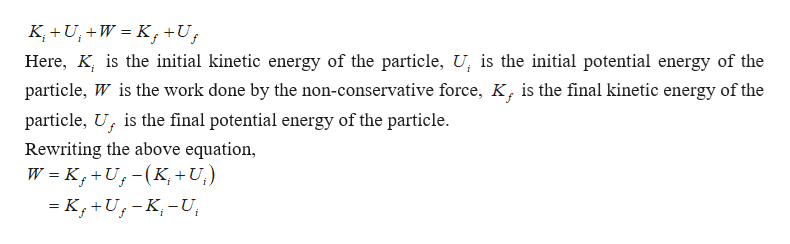# At time ti, the kinetic energy of a particle is41.1 J and its potential energy is 11.9 J. Atsome later time tf , its kinetic energy is 18.2 J and 34.8 J for potential energyIf the potential energy at time tf is 7.54 J,what is the work done by the nonconservativeforces acting on the particle?Answer in units of J.

Question
52 views

At time ti
, the kinetic energy of a particle is
41.1 J and its potential energy is 11.9 J. At
some later time tf , its kinetic energy is 18.2 J and 34.8 J for potential energy
If the potential energy at time tf is 7.54 J,
what is the work done by the nonconservative
forces acting on the particle?

check_circle

Step 1

The work-energy theorem gives,help_outlineImage TranscriptioncloseK U,+W K+U Here, K, is the initial kinetic energy of the particle, U, is the initial potential energy of the particle, W is the work done by the non-conservative force, K, is the final kinetic energy of the particle, U is the final potential energy of the particle Rewriting the above equation, W K, +U,-(K,+ U,) fullscreen
Step 2

Substitute the values in the above equation consider...

### Want to see the full answer?

See Solution

#### Want to see this answer and more?

Solutions are written by subject experts who are available 24/7. Questions are typically answered within 1 hour.*

See Solution
*Response times may vary by subject and question.
Tagged in

### Work,Power and Energy